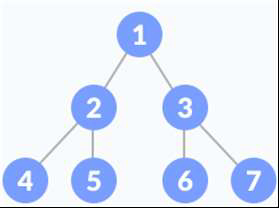# Golang program to define a binary tree

Example − In the given tree, the root node is 1, the root of its left sub tree is 2, and the root of its right sub tree is 3, ... so on.

Preorder Tree Traversal Output: 1, 2, 4, 5, 3, 6, 7.Approach to solve this problem

Step 1 − First, we’ll define the node structure.

Step 2 − In the main method, we would create the above tree.

Step 3 − Finally, we will perform the Preorder Tree Traversal.

## Example

Live Demo

package main
import "fmt"
type Node struct {
data int
left *Node
right *Node
}
func (root *Node)PreOrderTraversal(){
if root !=nil{
fmt.Printf("%d ", root.data)
root.left.PreOrderTraversal()
root.right.PreOrderTraversal()
}
return
}
func main(){
root := Node{1, nil, nil}
root.left = &Node{2, nil, nil}
root.right = &Node{3, nil, nil}
root.left.left = &Node{4, nil, nil}
root.left.right = &Node{5, nil, nil}
root.right.left = &Node{6, nil, nil}
root.right.right = &Node{7, nil, nil}
fmt.Printf("Pre Order Traversal of the given tree is: ")
root.PreOrderTraversal()
}

## Output

Pre Order Traversal of the given tree is − 1 2 4 5 3 6 7